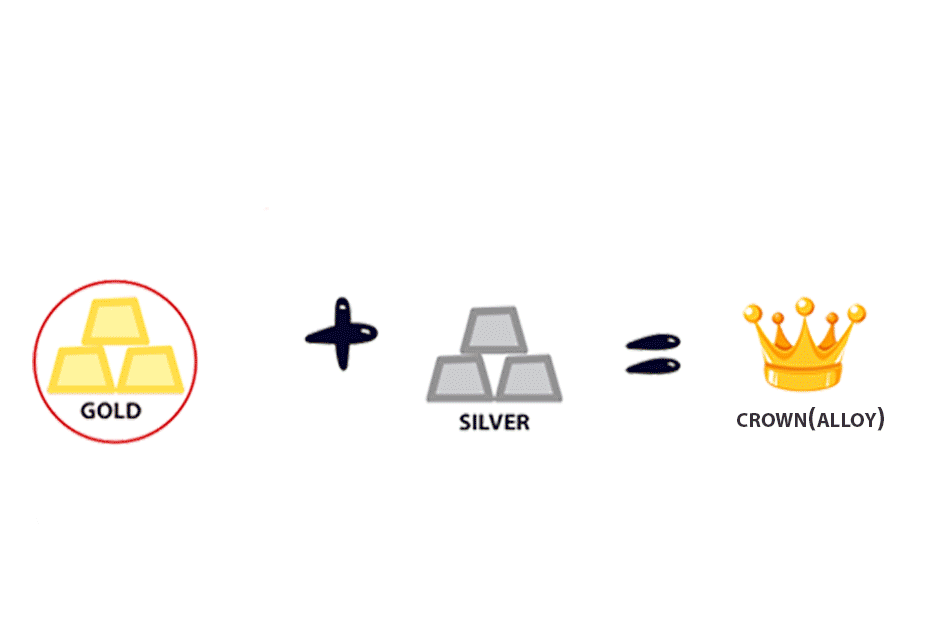#### PHYSICS | NECTA 2017 | Question 11

(a)(i) How does the increase of length and cross-section area of a conductor affect its resistance?

(b)(i) State the function of a circuit breaker in a wiring system.

(ii) Determine the ratio of resistance of wire A to that of wire B which are made up of the same material such that wire A has half the length and twice the diameter of wire B.

(c) An electric kettle contains 720 W heating units:

(i) What current does it take from 240 V mains?

(ii) How long will the kettle take to raise the temperature of 2 kg of water at 30°C to its boiling point?• SECTION 01

(a) How does the increase of length and cross-section area of a conductor affect its resistance?

Increase of length of conductor increases its resistance while increase of cross-sectional area of the conductor decreases its resistance

Resistance of conductor α (length / cross-sectional area)

• SECTION 02

(b)(i) State the function of a circuit breaker in a wiring system.

The main function of circuit breaker is to disconnect electricity when electric current exceeds the rated value of electric current.

(ii) Determine the ratio of resistance of wire A to that of wire B which are made up of the same material such that wire A has half the length and twice the diameter

of wire B.

Resistance,   R α l/A  , R = (kl)/A ... (i) hence RA= klA/AA = klA/d2A ... (ii) and R= klB/d2B …(iii)

(assume π/4 from cross-sectional area included within the constant …)

Resistance ratio = RA/ RB ... (iv), but lA = lB /2 = 0.5lB and dA = 2dB

Substitute equation (ii) and (iii) to equation (iv) we obtain ...

Resistance ratio = RA/ RB = (klA/d2A)/(klB/d2B) = (klA x d2B)/ (klB x d2A)(v)

Substitute the equivalent value of lA and dA to equation (v)

Resistance ratio = RA/ RB = [k0.5lB x d2B]/ [klB x (2d)2B]

Resistance ratio = [k0.5lB x d2B ]/[ klB x 4d2B ]= 0.5/4 = 1/8

Resistance ratio = 1/8

• SECTION 03

(c) An electric kettle contains 720 W heating units:

(i) What current does it take from 240 V mains?

P=VI

V = 240 v and P= 720W

I=P/V=(720W)/(240V)

I=3A

(ii) How long will the kettle take to raise the temperature of 2 kg of water at 30°C to its boiling point?

Data and Solution:

Temperature change = 30 to 100

Mass of water = 2Kg

Power equation:

P = E/t …. (i)

E=Pt

E = Specific Heat Capacity x Mass x Change in Temperature

E = SHC x M x ∆ . (ii)

Comparing equation (i) and equation (ii)

SHC x M x ∆ = Pt

t = [SHC x M x ∆]/P

t= [4200 x 2 x (100 - 30)]/720

t = 816.67sec = 13.6 min

Therefor it will take 13.6 min for the heater to raise temperature of water to boiling point.